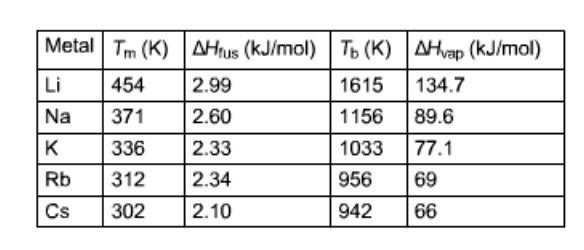# Problem: Using the following data, calculate ΔSfus (J•K -1•mol -1) and  ΔSvap (J•K -1•mol -1) for Na.

###### FREE Expert Solution
98% (295 ratings)
###### FREE Expert Solution
98% (295 ratings)###### Problem Details

Using the following data, calculate ΔSfus (J•K -1•mol -1) and  ΔSvap (J•K -1•mol -1) for Na.Frequently Asked Questions

What scientific concept do you need to know in order to solve this problem?

Our tutors have indicated that to solve this problem you will need to apply the Entropy concept. You can view video lessons to learn Entropy. Or if you need more Entropy practice, you can also practice Entropy practice problems.

What is the difficulty of this problem?

Our tutors rated the difficulty ofUsing the following data, calculate ΔSfus (J•K -1•mol -1) an...as medium difficulty.

How long does this problem take to solve?

Our expert Chemistry tutor, Dasha took 5 minutes and 16 seconds to solve this problem. You can follow their steps in the video explanation above.

What professor is this problem relevant for?

Based on our data, we think this problem is relevant for Professor Betancourt's class at TXSTATE.